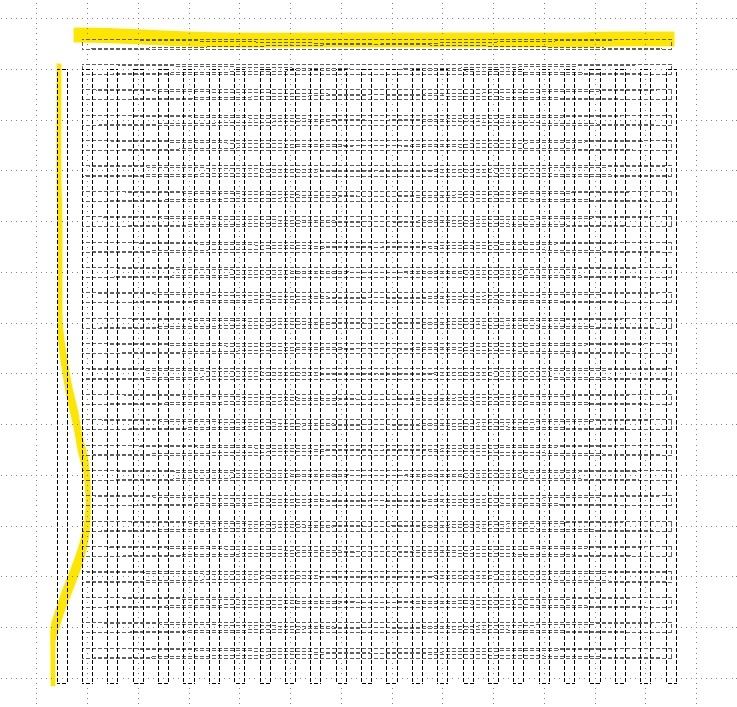# channel grid

not sure what's going on here trying too create an array of paths in the x,y
results are not as I expected`````` #Array Path Channels
ly = pya.CellView.active().layout()

ux = 600
uy = 600

for l in range(0, 25):

x = (l - 1) * 25.0
y = 0
uux  = x

l1 = ly.layer(1001,0)
ly.top_cell().shapes(l1).insert(pya.DBox(5,ux, -5.0, -5.0).moved(x, y))

for l in range(0, 25):

x = 0
y =  (l + 1) * 25.0

l1 = ly.layer(1000,0)
ly.top_cell().shapes(l1).insert(pya.DBox(-5 ,-5,uux, 5).moved(x, y))